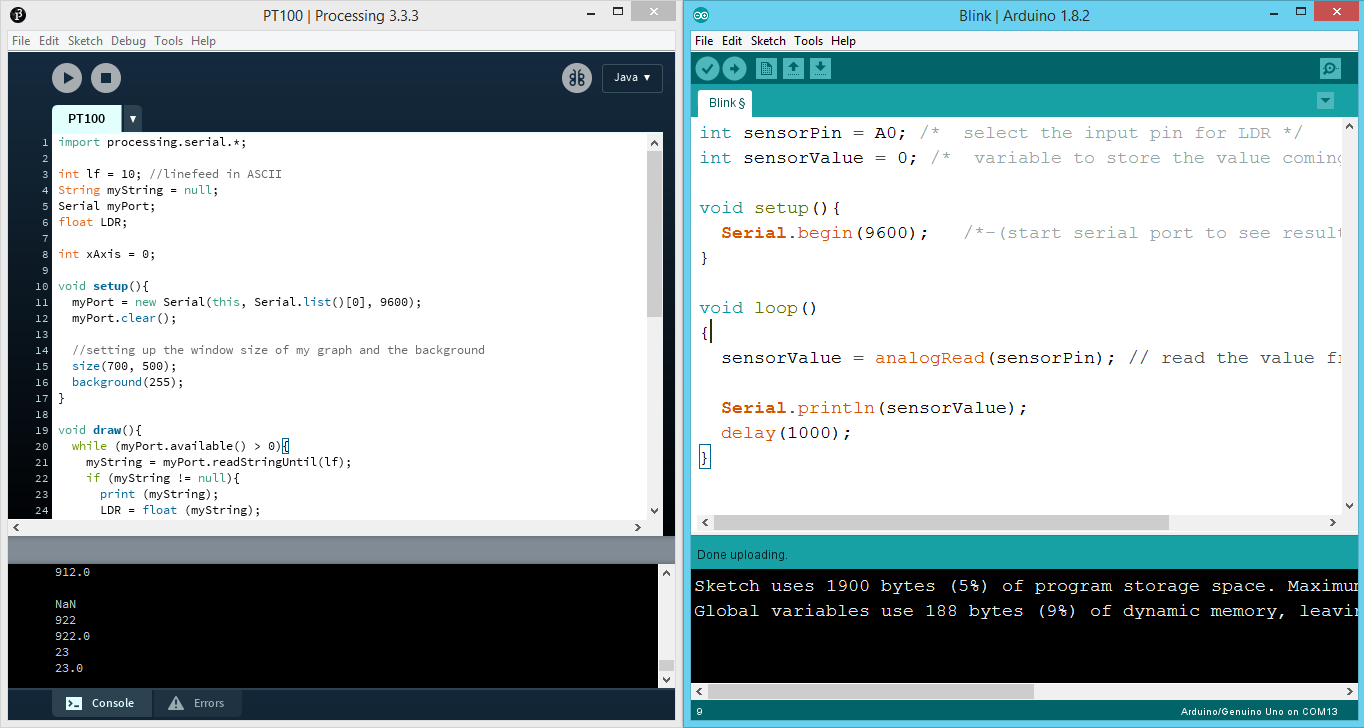# Interface and Application Programming

• Write an application that interfaces with an input and/or output device that you made, comparing as many tool options as possible.

• Interpret and implement design and programming protocols to create a Graphic User Interface (GUI).

For this week assigment I wanted to create something similar to what I have done in the Networking and Communications week, but instead of using Cayenne, I want to implement the same functionality by doing it myself

First of all, I want to make a button, which will switch my LED on and off.

Creating a two button graphical interface in Python to control a LED

I will use Python programming language to achieve this. The software which I will write, will communicate with the Arduino using Serial Communication

Here is the arduino code:

ArduinoIDE

```
int led = 13;
char mydata=0;
// the setup routine runs once when you press reset:
void setup() {
// initialize the digital pin as an output.
pinMode(led, OUTPUT);
Serial.begin(9600);
}

// the loop routine runs over and over again forever:
void loop() {

if (mydata=='1')
digitalWrite(led, HIGH);   // turn the LED on (HIGH is the voltage level)

if(mydata=='0')
digitalWrite(led, LOW);    // turn the LED off by making the voltage LOW
}
```

So first of all I define the pin where my LED is attached, Pin13 in my case. Next, I say that the initial value is 0 (LOW).

In the setup, I define the pin as OUTPUT, and set the baudrate for the communication - 9600

Next, I read from the serial. If there is "1" in the buffer, then switch the LED on, if there is "0" received, then switch the LED off

Basic stuff) Now lets get to Python!

Because I am using Ubuntu, python is already integrated. To start it, I open the terminal, and type `python`, and the shell should start

Before I start writing the code, I also have to instal one library called Tkinter. To do this on Ubuntu, I type in the teminal `sudo apt-get install python-tk`

This is the final sketch which I am using:

Python

```
import serial
from Tkinter import *

def led_on():
arduinoData.write(b'1')

def led_off():
arduinoData.write(b'0')

arduinoData = serial.Serial('/dev/ttyACM0',9600)
led_control_window= Tk()

btn=Button(led_control_window,text="led on",command=led_on, height=6, width=10)
btn2=Button(led_control_window,text="led off",command=led_off, height=6, width=10)

btn.pack()
btn2.pack()

```

First, I am importing the Serial library to communicate using serial, and import everything from the Tkinter library

Next, I create my function which will send the data to Arduino (LED on and off)

Then I define the port and the baudrate, as well as create a window from the Tk library

Next I create the two buttons, set up the dimensions of the button, and write the text

This is the final result:

The next thing which I want to make is read the values from the LDR, and plot them on a graph. Because I would like to experiment with different programming languages, I will be using Processing to achieve that!

Plotting LDR value using Processing on a graph

If you have a look at this picture, do you find anything familiar? Exactly! ArduinoIDE derived from Processing, that is why these two are very similarHere is the Arduino sketch which I am using:

Arduino IDE

```
int sensorPin = A0; /*  select the input pin for LDR */
int sensorValue = 0; /*  variable to store the value coming from the sensor */

void setup(){
Serial.begin(9600);   /*-(start serial port to see results )-*/
}

void loop()
{

Serial.println(sensorValue);
delay(1000);
}

```

Very simple code, but instead of using the Serial Monitor to print the LDR value, I use Processing to display the values and plot them on the Graph

Here is the Processing sketch:

Processing

```

import processing.serial.*;

int lf = 10; //linefeed in ASCII
String myString = null;
Serial myPort;
float LDR;

int xAxis = 0;

void setup(){
myPort = new Serial(this, Serial.list(), 9600);
myPort.clear();

//setting up the window size of my graph and the background
size(700, 500);
background(255);
}

void draw(){
while (myPort.available() > 0){
if (myString != null){
print (myString);
LDR = float (myString);
println(LDR);
LDR = map(LDR, 0, 1000, 0, height);
}
stroke(255, 0, 0);
line(xAxis, height - LDR, xAxis, height);
if(xAxis >= width){
xAxis = 0;
background(255);
}else{
xAxis++;
}
myPort.clear();
}
}

```

At first, I import the serial library, the same as in the Python sketch, and declare the LDR as float, define the port, and the X axis at zero

In the setup I read from the serial at the same port, and 9600 baudrate, clear the buffer, and set up the window size of my graph and the background color

And at final I draw my actual graph, indicating the dimensions, and the values which should be plotted

This is how everything works:

Arduino Code LED (.ino)

Python Code LED (.py)

Processing code (.pde)

Arduino Ploting Code (.ino)